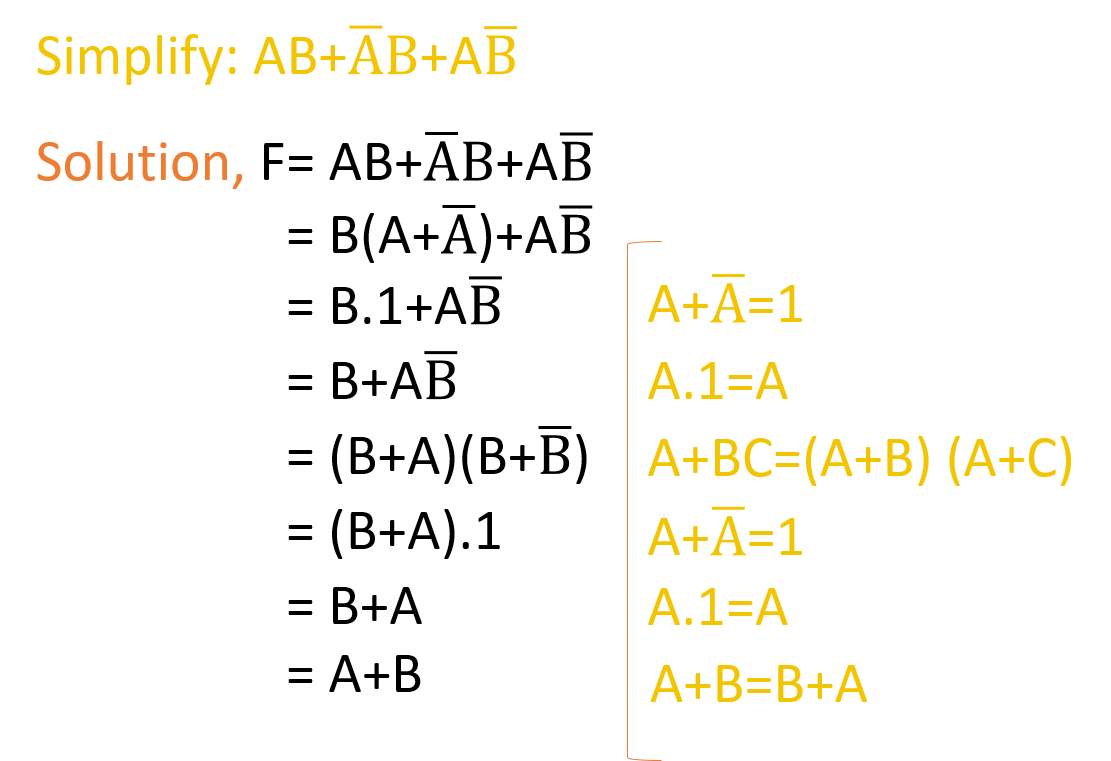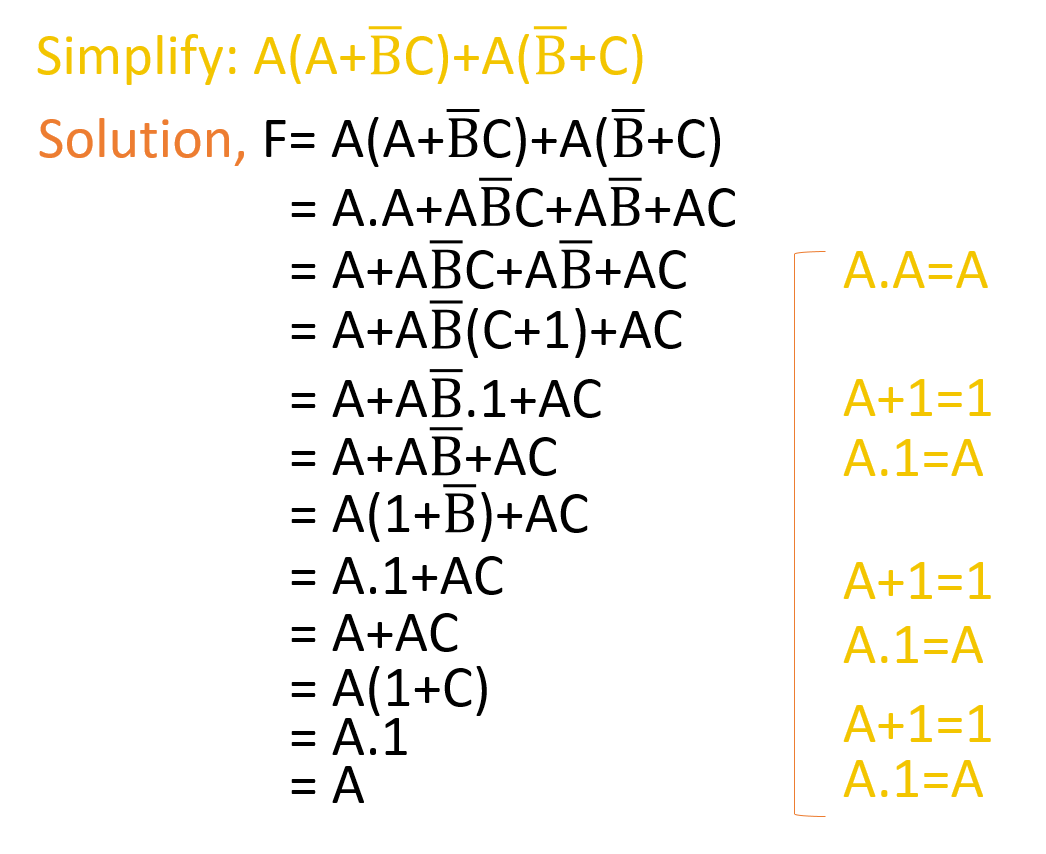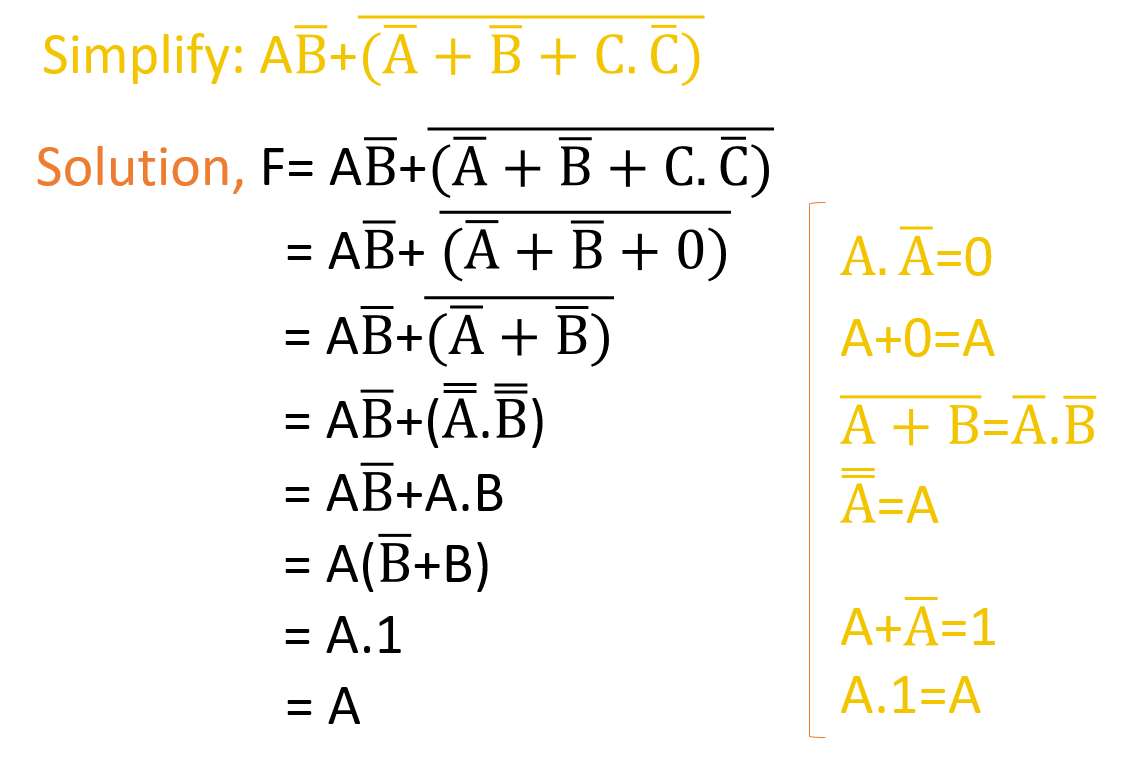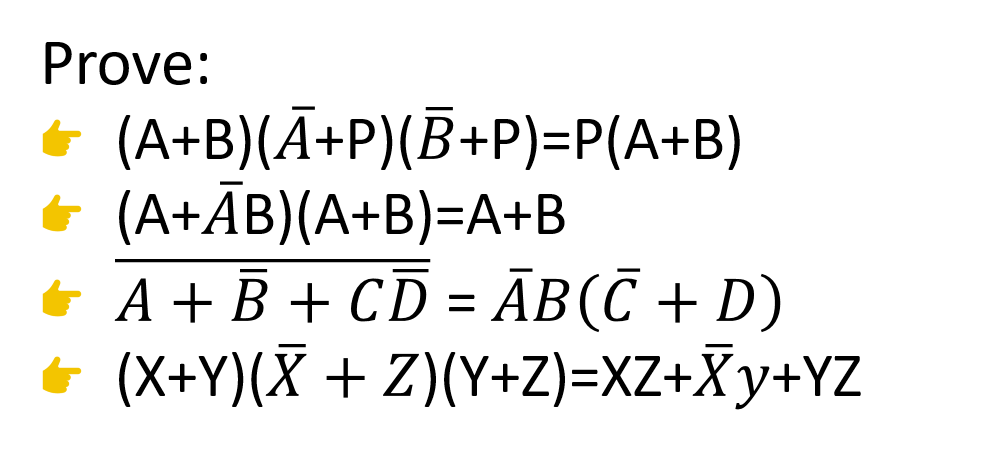# Third Chapter Lesson-10: Simplification of different Boolean Expressions.

## At the end of this lesson-

• 1. You will be able to describe the rules of simplifying the Boolean expressions.
• 2. You will be able to simplify the Boolean expressions.
• 3. You will be able to explain the importance of simplifying the Boolean expressions.

## Go for Bangla Version

The Boolean functions are implemented through the Logic Gates. In this case the number of logic operators in the function is less then the number of logic gates in the implementation is less. This makes implementation easier and saves money. Therefore, the Boolean functions are simplified with different Boolean theorem.

## The following rules or sequences are followed when Boolean expressions are simplified using Boolean theorem:

• First, we need to start simplifying from the left to the right of the expression.
• Second, The inner things of the parentheses “()” have to do.
• Third, the basic operations NOT, AND & OR of the Boolean algebra must be done respectively.
• Fourth, If no theorem can be applied to a specific step of simplification, then the equation can no longer be simplified.## Lesson Evaluation-

Knowledge Based Questions:

Comprehension Based Questions:

• b. Why Boolean expression is needed to simplify? Explain.